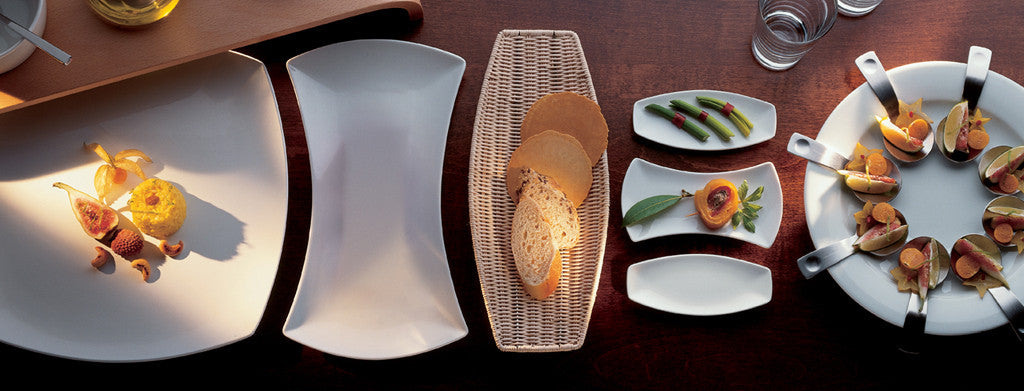Shopping Cart

### Refine

View all

#### Range# Crockery£2.84 each (ex VAT)£2.67 each (ex VAT)£0.89 each (ex VAT)£3.04 each (ex VAT)£15.27 each (ex VAT)£15.27 each (ex VAT)£16.51 each (ex VAT)£15.74 each (ex VAT)£0.98 each (ex VAT)£1.63 each (ex VAT)£16.28 each (ex VAT)£15.07 each (ex VAT)£2.70 each (ex VAT)£3.11 each (ex VAT)£2.55 each (ex VAT)£3.13 each (ex VAT)£3.06 each (ex VAT)£18.88 each (ex VAT)£15.15 each (ex VAT)£15.00 each (ex VAT)£19.75 each (ex VAT)£19.00 each (ex VAT)£16.51 each (ex VAT)£1.63 each (ex VAT)£3.17 each (ex VAT)£1.59 each (ex VAT)£1.32 each (ex VAT)£0.79 each (ex VAT)£3.07 each (ex VAT)£1.08 each (ex VAT)£2.93 each (ex VAT)£2.76 each (ex VAT)£2.57 each (ex VAT)£1.28 each (ex VAT)£1.56 each (ex VAT)£1.58 each (ex VAT)£16.99 each (ex VAT)£16.99 each (ex VAT)£16.99 each (ex VAT)£16.99 each (ex VAT)£16.99 each (ex VAT)£16.99 each (ex VAT)£16.29 each (ex VAT)£16.69 each (ex VAT)£16.99 each (ex VAT)£16.49 each (ex VAT)£16.13 each (ex VAT)£18.53